Published: 15 August 2015

# Dynamical analysis of fractional-order Mathieu equation

Shaofang Wen1
Yongjun Shen2
Xianghong Li3
Shaopu Yang4
Haijun Xing5
1Transportation Institute, Shijiazhuang Tiedao University, Shijiazhuang, China
2, 4, 5Department of Mechanical Engineering, Shijiazhuang Tiedao University, Shijiazhuang, China
3Department of Mathematics and Physics, Shijiazhuang Tiedao University, Shijiazhuang, China
Corresponding Author:
Yongjun Shen
Views 231

#### Abstract

The dynamical characteristics of Mathieu equation with fractional-order derivative is analytically studied by the Lindstedt-Poincare method and the multiple-scale method. The stability boundaries and the corresponding periodic solutions on these boundaries for the constant stiffness ${\delta }_{0}={n}^{2}$ ($n$ = 0, 1, 2, …), are analytically obtained. The effects of the fractional-order parameters on the stability boundaries and the corresponding periodic solutions, including the fractional coefficient and the fractional order, are characterized by the equivalent linear damping coefficient (ELDC) and the equivalent linear stiffness coefficient (ELSC). The comparisons between the transition curves on the boundaries obtained by the approximate analytical solution and the numerical method verify the correctness and satisfactory precision of the analytical solution. The following analysis is focused on the effects of the fractional parameters on the stability boundaries located in the $\delta -\epsilon$ plane. It is found that the increase of the fractional order $p$ could make the ELDC larger and ELSC smaller, which could result into the rightwards and upwards moving of the stability boundaries simultaneously. It could also be concluded the increase of the fractional coefficient ${K}_{1}$ would make the ELDC and ELSC larger, which could move the transition curves to the left and upwards at the same time. These results are very helpful to design, analyze or control this kind of system, and could present beneficial reference to the similar fractional-order system.

## 1. Introduction

In 1695, the French mathematician Hospital and German mathematician Leibniz put forward the concept of fractional-order calculus for the first time. In the following centuries, the theory about fractional-order calculus including the definition, characteristics, calculation of fractional-order calculus and the relationship with integer-order calculus, etc, had been developing rapidly and a series of meaningful results had been obtained [1-7].

In recent years, fractional-order calculus was paid more and more attention from researchers in different fields and became an international hot topic. A lot of mathematicians, physicists, chemists, dynamicists, and engineers in some relevant fields had applied this mathematical tool to solve the problems they met. For example, Shen and Yang et al. [8-11] investigated several linear and nonlinear fractional-order oscillators by averaging method, and found that the fractional-order derivatives had both damping and stiffness effects on the dynamical response in those oscillators. Gorenflo et al. , Jumarie , Ishteva et al.  and Agnieszka et al.  respectively studied the definitions and numerical methods of fractional-order calculus for Grünwald-Letnikov, Riemann-Liouville and Caputo. Chen and Zhu  reviewed the analytical methods and control strategies for quasi-integrable Hamiltonian systems with fractional-order derivatives, and pointed out some possible developing issues. They [17-20] also studied some nonlinear fractional-order system with different kinds of noise, and obtained some important statistic properties of the fractional-order system. Wang et al. [21-22] investigated a linear single degree-of-freedom oscillator with fractional-order derivative, and obtained the composition solution of its initial value problem without external excitation. Li et al.  discussed the properties of three kinds of fractional derivatives and sequential property of the Caputo derivative is also derived. Li et al. [24-26] had done a lot of researches in the mathematical theory of fractional-order calculus, and also established some efficient numerical algorithms. Wahi and Chatterjee  studied a special linear single degree-of-freedom oscillator with fractional-order derivative by averaging method, and analyzed the effects of the fractional-orders derivative. Xu et al.  used Lindstedt-Poincaré method and the multiple-scale approach to investigate fractional-order Duffing oscillator subjected to random excitation.

In addition to the theoretical research, fractional calculus had also been used to solve engineering problems. Comparing with the traditional integer-order system, the fractional-order system is much closer to the real nature of the world, and has more advantages, such as strong ability of anti-noise, good robustness, high control precision and so on. Accordingly fractional-order calculus has the significant value for the engineering field.

The well-known Mathieu equation, first introduced by Mathieu  when he studied the vibration of elliptical membranes in 1868, is a linear differential equation with periodic coefficients. Mathieu equation had been applied in physics and engineering fields, and many complex dynamical properties had been found herein. In recent years, many scholars had studied the Mathieu equation and found that the fractional-order system could generate different dynamical properties from the integer-order system. For example, the chaotic behaviors of a nonlinear damped Mathieu system were investigated by applying numerical integration method . Ebaid et al.  considered the fractional-order calculus model of damped Mathieu equation and obtained the approximate analytical solution by using two different methods. The transition curves separating the stable and unstable regions in the fractional-order Mathieu equation had been studied by the method of harmonic balance [32-33], and Leung et al. also investigated a general version of fractional-order Mathieu-Duffing equation by harmonic balance method.

In this paper, the fractional-order Mathieu equation with linear damping is studied by the Lindstedt-Poincaré (L-P) method coupled with the multiple-scale method, and the effects of the fractional parameters on the dynamical properties of the fractional-order Mathieu equation are analyzed in detail. The paper is organized as follow. Section 2 presents the stability boundaries and the corresponding approximately analytical periodic solutions on these boundaries for the constant stiffness ${\delta }_{0}={n}^{2}$ ($n=$ 0, 1, 2, …), where the effects of the fractional-order derivative on the system damping and stiffness are formulated as equivalent linear damping coefficient (ELDC) and equivalent linear stiffness coefficient (ELSC). In section 3, the comparisons between the boundaries obtained by the approximate analytical solution and the numerical method verify the correctness and satisfactory precision of the analytical solution. The following analysis is focused on the effects of the fractional parameters on the stability boundaries in the $\delta -\epsilon$ plane. At last the detail results are summarized and the conclusions are made.

## 2. Fractional-order Mathieu equation

In this paper, we shall consider the fractional-order Mathieu equation as:

1
$\stackrel{¨}{x}\left(t\right)+2\mathrm{\zeta }\stackrel{˙}{x}\left(t\right)+\left(\mathrm{\delta }+2\mathrm{\epsilon }\mathrm{c}\mathrm{o}\mathrm{s}2t\right)x\left(t\right)+{K}_{1}{D}^{p}\left[x\left(t\right)\right]=0,\mathrm{}\mathrm{}\mathrm{}\mathrm{}\left|\epsilon \right|\ll 1,\mathrm{}\mathrm{}\mathrm{}0<\zeta \ll 1,$

where $2\zeta$, $\delta$, and $2\epsilon \mathrm{c}\mathrm{o}\mathrm{s}2t$ are the system linear damping coefficient, constant stiffness coefficient and the periodic time-varying coefficient respectively. ${D}^{p}\left[x\left(t\right)\right]$ is the $p$-order derivative of $x\left(t\right)$ to $t$ with the fractional coefficient ${K}_{1}$ (${K}_{1}>0$) and the fractional order $p$ ($0\le p\le 1$). There are several definitions for fractional-order derivative, such as Grünwald-Letnikov, Riemann-Liouville and Caputo definitions [1-7]. Here Caputo’s definition is adopted with the form as:

2
${D}^{p}\left[x\left(t\right)\right]=\frac{1}{\mathrm{\Gamma }\left(1-p\right)}{\int }_{0}^{t}\frac{{x}^{\mathrm{\text{'}}}\left(u\right)}{{\left(t-u\right)}^{p}}du,$

where $\mathrm{\Gamma }\left(y\right)$ is Gamma function satisfying $\mathrm{\Gamma }\left(y+1\right)=y\mathrm{\Gamma }\left(y\right)$.

## 3. Approximately analytical solution and the stability boundaries

The approximately analytical solution and the stability boundaries are determined under the conditions of small damping coefficient. Using the parameters transformation:

$\zeta =\epsilon \mu ,\mu =O\left(1\right),{K}_{1}=\epsilon k,k=O\left(1\right).$

Eq. (1) becomes:

3
$\stackrel{¨}{x}\left(t\right)+2\epsilon \mu \stackrel{˙}{x}\left(t\right)+\left(\delta +2\epsilon \mathrm{c}\mathrm{o}\mathrm{s}2t\right)x\left(t\right)+\epsilon k{D}^{p}\left[x\left(t\right)\right]=0.$

Introducing the fast and slow time scales as follows:

${T}_{r}\stackrel{\mathrm{d}\mathrm{e}\mathrm{f}}{=}{\epsilon }^{r}t,r=0,\mathrm{}1,\mathrm{}2,\dots ,$

and the differentiation operators:

4
${D}_{0}=\frac{\partial }{\partial {T}_{0}},{D}_{1}=\frac{\partial }{\partial {T}_{1}},{D}_{2}=\frac{\partial }{\partial {T}_{2}}$

the ordinary derivative can be transformed into the partial derivatives  as follows:

5
$\begin{array}{c}\frac{d}{dt}={D}_{0}+\epsilon {D}_{1}+\dots ,\end{array}$
$\frac{{d}^{2}}{d{t}^{2}}={D}_{0}^{2}+2\epsilon {D}_{0}{D}_{1}+\dots .$

The approximate solution of Eq. (3) can be written as:

6a
$x={x}_{0}\left({T}_{0},{T}_{1},{T}_{2}\right)+\epsilon {x}_{1}\left({T}_{0},{T}_{1},{T}_{2}\right)+{\epsilon }^{2}{x}_{2}\left({T}_{0},{T}_{1},{T}_{2}\right)+\dots .$

Applying the modified L-P method, we introduce the following variable transformation [34-35]:

6b
$\delta ={\delta }_{0}+\epsilon {\delta }_{1}+{\epsilon }^{2}{\delta }_{2}+{\epsilon }^{3}{\delta }_{3}+\dots .$

Substituting Eq. (5), Eq. (6a), and Eq. (6b) into Eq. (3) and separating the terms in the yielded equation based on the power of $\epsilon$, we derive the following equations:

7
$O\left({\mathrm{\epsilon }}^{0}\right):{D}_{0}^{2}{x}_{0}+\mathrm{\delta }{}_{0}{}^{\mathrm{}}\mathrm{}{x}_{0}=0,$
8
$O\left({\epsilon }^{1}\right):{D}_{0}^{2}{x}_{1}+\delta {}_{0}{}^{}{x}_{1}=-2{D}_{0}{D}_{1}{x}_{0}-2\mu {D}_{0}{x}_{0}-\delta {}_{1}{}^{}{x}_{0}-2cos2T{}_{0}{}^{}{x}_{0}-k{D}_{0}^{p}{x}_{0},$
9
$O\left({\epsilon }^{2}\right):{D}_{0}^{2}{x}_{2}+\delta {}_{0}{}^{}{x}_{2}=-2{D}_{0}{D}_{2}{x}_{0}-2{D}_{0}{D}_{1}{x}_{1}-{D}_{1}^{2}{x}_{0}-2\mu {D}_{0}{x}_{1}$
$-2\mu {D}_{1}{x}_{0}-\delta {}_{2}{}^{}{x}_{0}-\delta {}_{1}{}^{}{x}_{1}-2\mathrm{c}\mathrm{o}\mathrm{s}2T{}_{0}{}^{}{x}_{1}-k{D}_{0}^{p}{x}_{1}-k{D}_{1}^{p}{x}_{0}.$

The solution of Eq. (7) can be written as:

10
${x}_{0}=A\left({T}_{1},{T}_{2}\right){e}^{i\sqrt{{\delta }_{0}}{T}_{0}}+cc,$

where $cc$ denotes the complex conjugate of the preceding term. The approximately analytical solution and the boundary will be presented in the following parts according to the different constant stiffness coefficient ${\delta }_{0}$.

### 3.1. ${\mathbit{\delta }}_{0}=0$

According to Eq. (7) and Eq. (10), the solution can be written as:

11
${x}_{0}=a=\mathrm{c}\mathrm{o}\mathrm{n}\mathrm{s}\mathrm{t},$

where $a$ is an arbitrary constant and determined by the initial condition. Substituting Eq. (11) into Eq. (8) one could obtain:

${D}_{0}^{2}{x}_{1}=-a\left({\delta }_{1}+2\mathrm{c}\mathrm{o}\mathrm{s}2{T}_{0}\right).$

In order to eliminate the secular terms in ${x}_{1}$, one must put:

12a
${\delta }_{1}=0.$

The solution of Eq. (8) is:

12b
${x}_{1}=\frac{a}{2}\mathrm{c}\mathrm{o}\mathrm{s}2{T}_{0}.$

Based on the formula for fractional derivative , ${D}^{p}{e}^{i\lambda t}$ could be approximately reduced to:

13
${D}^{p}{e}^{i\lambda t}=\left(i\lambda {\right)}^{p}{e}^{i\lambda t}.$

According to Eq. (13) and the Euler formula, one can obtain:

14
$k{D}_{0}^{p}{x}_{1}=k{D}_{0}^{p}\left(\frac{a}{2}\mathrm{c}\mathrm{o}\mathrm{s}2{T}_{0}\right)=\frac{a}{2}k{D}_{0}^{p}\left(\frac{{e}^{i2{T}_{0}}+{e}^{-i2{T}_{0}}}{2}\right)=\frac{ka}{4}\left[\left(2i{\right)}^{p}{e}^{i2{T}_{0}}+\left(-2i{\right)}^{p}{e}^{-i2{T}_{0}}].$

Substituting Eq. (11), Eq. (12), Eq. (13) and Eq. (14) into Eq. (9), we obtain:

15
${D}_{0}^{2}{x}_{2}=-a\left({\delta }_{2}+\frac{1}{2}\right)+2\mu a\mathrm{s}\mathrm{i}\mathrm{n}2{T}_{0}-\frac{a}{2}\mathrm{c}\mathrm{o}\mathrm{s}4{T}_{0}-\frac{1}{4}ka\left[\left(2i{\right)}^{p}{e}^{i2{T}_{0}}+\left(-2i{\right)}^{p}{e}^{-i2{T}_{0}}].$

To eliminate the secular terms, we arrive at:

16
$-a\left({\delta }_{2}+\frac{1}{2}\right)=0,$

and:

16a
${\delta }_{2}=-\frac{1}{2}.$

The solution of Eq. (9) is derived as:

16b
${x}_{2}=-\frac{\mu a}{2}\mathrm{s}\mathrm{i}\mathrm{n}2{T}_{0}+\frac{a}{32}\mathrm{c}\mathrm{o}\mathrm{s}4{T}_{0}+\frac{1}{16}ka\left(2i{\right)}^{p}{e}^{i2{T}_{0}}+\frac{1}{16}ka\left(-2i{\right)}^{p}{e}^{-i2{T}_{0}},$

where the formula:

17
${i}^{p}=\left({e}^{\frac{i\pi }{2}}{\right)}^{p}={e}^{\frac{ip\pi }{2}}=\mathrm{c}\mathrm{o}\mathrm{s}\frac{p\pi }{2}+i\mathrm{s}\mathrm{i}\mathrm{n}\frac{p\pi }{2},$

is used in Eq. (16b). One can get another form of Eq. (16b):

18
$x{}_{2}{}^{}=\frac{a}{2}\left[-\mu \mathrm{s}\mathrm{i}\mathrm{n}2{T}_{0}+\frac{{2}^{p}k}{4}\mathrm{c}\mathrm{o}\mathrm{s}\left(\frac{p\pi }{2}+2{T}_{0}\right)\right]+\frac{a}{32}\mathrm{c}\mathrm{o}\mathrm{s}4{T}_{0}+O\left({\epsilon }^{3}\right).$

Substituting Eq. (12a) and Eq. (16a) into Eq. (6b), the transition curve of the stability boundary near to ${\delta }_{0}=0$ is given by:

19
$\delta \left(\epsilon \right)=-\frac{{\epsilon }^{2}}{2}+O\left({\epsilon }^{3}\right).$

Substituting Eq. (11), Eq. (12b) and Eq. (18) into Eq. (6a), one could obtain the corresponding periodic solution with period $\pi$ on this curve:

20
$x=a+\frac{\epsilon a}{2}\left\{\left[1+\frac{{2}^{p}K{}_{1}{}^{\mathrm{}}\mathrm{}}{4}\mathrm{c}\mathrm{o}\mathrm{s}\left(\frac{p\mathrm{\pi }}{2}\right)\right]\mathrm{c}\mathrm{o}\mathrm{s}2t-\left[\zeta +\frac{{2}^{p}K{}_{1}{}^{}}{4}\mathrm{s}\mathrm{i}\mathrm{n}\left(\frac{p\pi }{2}\right)\right]\mathrm{s}\mathrm{i}\mathrm{n}2t\right\}$
$\mathrm{}\mathrm{}\mathrm{}\mathrm{}\mathrm{}\mathrm{}+\frac{{\mathrm{\epsilon }}^{2}a}{32}\mathrm{c}\mathrm{o}\mathrm{s}4t+O\left({\mathrm{\epsilon }}^{3}\right).$

From Eq. (19), we can know that the result of the transition curve is independent of the fractional coefficient ${K}_{1}$ and the fractional order $p$. In order to analyze the effects of the fractional-order derivative, we must obtain the third-order approximate solution:

21
$O\left({\mathrm{\epsilon }}^{3}\right):{D}_{0}^{2}{x}_{3}+\mathrm{\delta }{}_{0}{}^{\mathrm{}}\mathrm{}{x}_{3}=-2{D}_{1}{D}_{0}{x}_{2}-2{D}_{1}{D}_{2}{x}_{0}-{D}_{1}^{2}{x}_{1}-2{D}_{2}{D}_{0}{x}_{1}-2\mathrm{c}\mathrm{o}\mathrm{s}2T{}_{0}{}^{\mathrm{}}\mathrm{}{x}_{2}$
$-\delta {}_{3}{}^{}{x}_{0}-\delta {}_{2}{}^{}{x}_{1}-\delta {}_{1}{}^{}{x}_{2}-2\mu {D}_{0}{x}_{2}-2\mu {D}_{1}{x}_{1}-2\mu {D}_{2}{x}_{0}$
$-k{D}_{0}^{p}{x}_{2}-k{D}_{1}^{p}{x}_{1}-k{D}_{2}^{p}{x}_{0}.$

As the similar procedure, we arrive at:

$-{\delta }_{3}a-\frac{{2}^{p}ka}{8}\mathrm{c}\mathrm{o}\mathrm{s}\left(\frac{p\pi }{2}\right)=0$

and:

22
${\delta }_{3}=-\frac{{2}^{p}k}{8}\mathrm{c}\mathrm{o}\mathrm{s}\left(\frac{p\pi }{2}\right),$

based on the eliminating procedure for the secular term.

In the case ${\delta }_{0}=0$, the third-order approximate solution could be established as:

23
${\mathrm{\Delta }}_{0}=-\frac{{\mathrm{\epsilon }}^{2}}{2}+O\left({\mathrm{\epsilon }}^{4}\right),$

where ${\mathrm{\Delta }}_{0}=\delta +{\epsilon }^{2}\frac{{2}^{p}{K}_{1}}{8}\mathrm{c}\mathrm{o}\mathrm{s}\left(\frac{p\pi }{2}\right)$, which is defined as the equivalent linear stiffness coefficient (ELSC) in the case ${\delta }_{0}=0$.

### 3.2. ${\mathbit{\delta }}_{0}=\text{1}$

In the case ${\delta }_{0}=\text{1}$, the solution of Eq. (7) can be written as:

24
${x}_{0}=A{e}^{i{T}_{0}}+cc.$

Using Eq. (13), one can get:

25
$k{D}_{0}^{p}{x}_{0}=k{D}_{0}^{p}\left(A{e}^{i{T}_{0}}+cc\right)=k{i}^{p}A{e}^{i{T}_{0}}+cc.$

Substituting Eq. (24) and Eq. (25) into Eq. (8), we can obtain:

26
${D}_{0}^{2}{x}_{1}+{x}_{1}=\left(-2i{D}_{1}A-2i\mu A-{\delta }_{1}A-\stackrel{-}{A}-k{i}^{p}A\right){e}^{i{T}_{0}}-A{e}^{i3{T}_{0}}+cc.$

After eliminating the secular terms, we arrive at:

27
$-2i{D}_{1}A-2i\mu A-{\delta }_{1}A-\stackrel{-}{A}-k{i}^{p}A=0$

where ${D}_{1}A$ stands for the derivative with the respect to the slow time scale ${T}_{1}$, and $\stackrel{-}{A}$ means the conjugate of $A$. In this paper, we want to obtain the periodic solutions, so that the hypothesis ${D}_{1}A=$0 is reasonable.

Substituting $A=a/2-b/2i$ and Eq. (17) into Eq. (27), and after separating the real and imaginary parts of Eq. (27), we obtain:

28
$\left\{\begin{array}{l}\left[1+{\delta }_{1}+k\mathrm{c}\mathrm{o}\mathrm{s}\left(\frac{p\pi }{2}\right)\right]a+\left[2\mu +k\mathrm{s}\mathrm{i}\mathrm{n}\left(\frac{p\pi }{2}\right)\right]b=0,\\ \left[2\mu +k\mathrm{s}\mathrm{i}\mathrm{n}\left(\frac{p\pi }{2}\right)\right]a+\left[1-{\delta }_{1}-k\mathrm{c}\mathrm{o}\mathrm{s}\left(\frac{p\pi }{2}\right)\right]b=0.\end{array}\right\$

The necessary conditions for existing nonzero solution about $\left(a,b\right)$ in Eq. (28) is:

29
$\mathrm{d}\mathrm{e}\mathrm{t}\left[\begin{array}{cc}1+{\delta }_{1}+k\mathrm{c}\mathrm{o}\mathrm{s}\left(\frac{p\pi }{2}\right)& 2\mu +k\mathrm{s}\mathrm{i}\mathrm{n}\left(\frac{p\pi }{2}\right)\\ 2\mu +k\mathrm{s}\mathrm{i}\mathrm{n}\left(\frac{p\pi }{2}\right)& 1-{\delta }_{1}-k\mathrm{c}\mathrm{o}\mathrm{s}\left(\frac{p\pi }{2}\right)\end{array}\right]=0.$

That is:

30
$\left[1+{\delta }_{1}+k\mathrm{c}\mathrm{o}\mathrm{s}\left(\frac{p\pi }{2}\right)\right]\left[1-{\delta }_{1}-k\mathrm{c}\mathrm{o}\mathrm{s}\left(\frac{p\pi }{2}\right)\right]-{\left[2\mu +k\mathrm{s}\mathrm{i}\mathrm{n}\left(\frac{p\pi }{2}\right)\right]}^{2}=0.$

Then, we can get:

31
${\delta }_{1}=±\sqrt{1-{\left[2\mu +k\mathrm{s}\mathrm{i}\mathrm{n}\left(\frac{p\pi }{2}\right)\right]}^{2}}-k\mathrm{c}\mathrm{o}\mathrm{s}\left(\frac{p\pi }{2}\right).$

In this case, the particular solution of Eq. (8) is:

32
${x}_{1}=\frac{A}{8}{e}^{i3{T}_{0}}+cc.$

Substituting Eq. (24) and Eq. (32) into Eq. (9), we can obtain:

33
${D}_{0}^{2}{x}_{2}+{x}_{2}=\left(-2i{D}_{2}A-{D}_{1}^{2}A-2\mu {D}_{1}A-{\delta }_{2}A-\frac{A}{8}-k{D}_{1}^{p}A\right){e}^{i{T}_{0}}$
$+\left[-\frac{3}{4}i{D}_{1}A-\frac{3}{4}i\mu A-\frac{{\delta }_{1}A}{8}-\frac{\left(3i{\right)}^{p}kA}{8}\right]{e}^{i3{T}_{0}}-\frac{A}{8}{e}^{i5{T}_{0}}+cc.$

After eliminating the secular terms, one can get:

34
$-2i{D}_{2}A-{D}_{1}^{2}A-2\mu {D}_{1}A-{\delta }_{2}A-\frac{A}{8}-k{D}_{1}^{p}A=0.$

As the similar approach, we can suppose ${D}_{1}A=0$, ${D}_{2}A=0$ and ${D}_{1}^{p}A=0$. From Eq. (34) we can obtain:

35
${\delta }_{2}=-\frac{1}{8}.$

In this case, the particular solution of Eq. (9) is:

36
${x}_{2}=\left(i\frac{3\mu A}{32}+\frac{{\delta }_{1}A}{64}+{i}^{p}\frac{{3}^{p}kA}{64}\right){e}^{i3{T}_{0}}+\frac{A}{192}{e}^{i5{T}_{0}}+cc.$

Substituting Eq. (31) and Eq. (35) into Eq. (6b), the two transition curves emanating from ${\delta }_{0}=\text{1}$ are given by:

37
${\mathrm{\Delta }}_{1}=1±\sqrt{{\epsilon }^{2}-{C}_{1}^{2}}-\frac{1}{8}{\epsilon }^{2}+O\left({\epsilon }^{3}\right),$

where ${\mathrm{\Delta }}_{1}=\delta +{K}_{1}\mathrm{c}\mathrm{o}\mathrm{s}\left(p\pi /2\right)$ and ${C}_{1}=2\zeta +{K}_{1}\mathrm{s}\mathrm{i}\mathrm{n}\left(p\pi /2\right)$. Here ${\mathrm{\Delta }}_{1}$ and ${C}_{1}$ are defined as the equivalent linear stiffness coefficient and the equivalent linear damping coefficient respectively in the case ${\delta }_{0}=\text{1}$.

Substituting Eq. (24), Eq. (32) and Eq. (36) into Eq. (6a), one could obtain the corresponding periodic solution with period 2$\pi$ on these curves:

38
$x=A{e}^{i{T}_{0}}+\epsilon \frac{A}{8}{e}^{i3{T}_{0}}+{\epsilon }^{2}\left(i\frac{3\mu A}{32}+\frac{{\delta }_{1}A}{64}+{i}^{p}\frac{{3}^{p}kA}{64}\right){e}^{i3{T}_{0}}+{\epsilon }^{2}\frac{A}{192}{e}^{i5{T}_{0}}+cc+O\left({\epsilon }^{3}\right).$

Substituting Eq. (17), Eq. (37) and $A=a/2-b/2i$ into Eq. (38), one can get another form of Eq. (38) with the original parameters:

39
$x=a\mathrm{c}\mathrm{o}\mathrm{s}t+b\mathrm{s}\mathrm{i}\mathrm{n}t+\frac{\epsilon }{8}\left\{\left[\left(1+\frac{\delta }{8}\right)a+\frac{3\zeta b}{4}\right]\mathrm{c}\mathrm{o}\mathrm{s}3t+\left[\left(1+\frac{\delta }{8}\right)b-\frac{3\zeta a}{4}\right]\mathrm{s}\mathrm{i}\mathrm{n}3t\right\}$
$+\frac{{3}^{p}{K}_{1}\epsilon }{64}\left\{\left[\mathrm{c}\mathrm{o}\mathrm{s}\left(\frac{p\pi }{2}\right)a+\mathrm{s}\mathrm{i}\mathrm{n}\left(\frac{p\pi }{2}\right)b\right]\mathrm{c}\mathrm{o}\mathrm{s}3t+\left[\mathrm{c}\mathrm{o}\mathrm{s}\left(\frac{p\pi }{2}\right)b-\mathrm{s}\mathrm{i}\mathrm{n}\left(\frac{p\pi }{2}\right)a\right]\mathrm{s}\mathrm{i}\mathrm{n}3t\right\}$
$+\frac{\epsilon }{64}\left[±\sqrt{{\epsilon }^{2}-{{C}_{1}}^{2}}-{\mathrm{\Delta }}_{1}\right]\left(a\mathrm{c}\mathrm{o}\mathrm{s}3t+b\mathrm{s}\mathrm{i}\mathrm{n}3t\right)+\frac{{\epsilon }^{2}}{192}\left(a\mathrm{c}\mathrm{o}\mathrm{s}5t+b\mathrm{s}\mathrm{i}\mathrm{n}5t\right)+O\left({\epsilon }^{3}\right).$

### 3.3. ${\mathbit{\delta }}_{0}=\text{4}$

In order to analyze the effect of damping ratio near $\delta =\text{4}$, one should assumed $\zeta =O\left({\epsilon }^{2}\right)$. Using the transformation:

$\zeta ={\epsilon }^{2}\stackrel{^}{\mu },\stackrel{^}{\mu }=O\left(1\right),{K}_{1}={\epsilon }^{2}\stackrel{^}{k},\stackrel{^}{k}=O\left(1\right).$

Eq. (1) becomes:

40
$\stackrel{¨}{x}\left(t\right)+2{\epsilon }^{2}\stackrel{^}{\mu }\stackrel{˙}{x}\left(t\right)+\left(\delta +2\epsilon cos2t\right)x\left(t\right)+{\epsilon }^{2}\stackrel{^}{k}{D}^{p}\left[x\left(t\right)\right]=0.$

Eq. (8) and Eq. (9) become:

41
$O\left({\epsilon }^{1}\right):{D}_{0}^{2}{x}_{1}+{\delta }_{0}{x}_{1}=-2{D}_{0}{D}_{1}{x}_{0}-{\delta }_{1}{x}_{0}-2\mathrm{c}\mathrm{o}\mathrm{s}2{T}_{0}{x}_{0},$
42
$O\left({\epsilon }^{2}\right):{D}_{0}^{2}{x}_{2}+\delta {}_{0}{}^{}{x}_{2}=-2{D}_{0}{D}_{2}{x}_{0}-2{D}_{0}{D}_{1}{x}_{1}-{D}_{1}^{2}{x}_{0}-2\stackrel{^}{\mu }{D}_{0}{x}_{0}-\stackrel{^}{k}{D}_{0}^{p}{x}_{0}$
$-\delta {}_{2}{}^{}{x}_{0}-\delta {}_{1}{}^{}{x}_{1}-2\mathrm{c}\mathrm{o}\mathrm{s}2{T}_{0}{x}_{1}.$

In the case ${\delta }_{0}=\text{4}$, the solution of Eq. (7) can be written as:

43
${x}_{0}=A{e}^{i2{T}_{0}}+cc.$

Substituting Eq. (43) into Eq. (41), we get:

44
${D}_{0}^{2}{x}_{1}+4{x}_{1}=\left(-4i{D}_{1}A-{\delta }_{1}A\right){e}^{i2{T}_{0}}-A{e}^{i4{T}_{0}}+cc.$

To eliminate the secular terms, we arrive at $-4i{D}_{1}A-{\delta }_{1}A=0$. Due to ${D}_{1}^{p}A=0$, one can get ${\delta }_{1}=0$. The solution of Eq. (41) is:

45
${x}_{1}=\frac{A}{12}{e}^{i4{T}_{0}}-\frac{A}{4}+cc.$

Substituting Eq. (43) and Eq. (45) into Eq. (42), we get:

46
${D}_{0}^{2}{x}_{2}+4{x}_{2}=\left(-4i{D}_{2}A-{D}_{1}^{2}A-4i\stackrel{^}{\mu }A-{2}^{p}{i}^{p}\stackrel{^}{k}A-{\delta }_{2}A+\frac{A}{6}-\frac{\stackrel{-}{A}}{4}\right){e}^{i2{T}_{0}}$
$-\frac{2i{D}_{1}A}{3}{e}^{i4{T}_{0}}-\frac{A}{12}{e}^{i6{T}_{0}}+cc.$

To eliminate the secular terms, we arrive at:

$-4i{D}_{2}A-{D}_{1}^{2}A-4i\stackrel{^}{\mu }A-{2}^{p}{i}^{p}\stackrel{^}{k}A-{\delta }_{2}A+\frac{A}{6}-\frac{\stackrel{-}{A}}{4}=0.$

As the same procedure in the case ${\delta }_{0}=\text{1}$, we get:

$\mathrm{d}\mathrm{e}\mathrm{t}\left[\begin{array}{cc}-\left[{2}^{p}\stackrel{^}{k}\mathrm{c}\mathrm{o}\mathrm{s}\left(\frac{p\pi }{2}\right)\right]+{\delta }_{2}+\frac{1}{12}& -\left[{2}^{p}\stackrel{^}{k}\mathrm{s}\mathrm{i}\mathrm{n}\left(\frac{p\pi }{2}\right)+4\stackrel{^}{\mu }\right]\\ -\left[{2}^{p}\stackrel{^}{k}\mathrm{s}\mathrm{i}\mathrm{n}\left(\frac{p\pi }{2}\right)+4\stackrel{^}{\mu }\right]& \left[{2}^{p}\stackrel{^}{k}\mathrm{c}\mathrm{o}\mathrm{s}\left(\frac{p\pi }{2}\right)+{\delta }_{2}-\frac{5}{12}\right]\end{array}\right]=0.$

That will result into:

47
${\delta }_{2}=\frac{1}{6}-{2}^{p}\stackrel{^}{k}\mathrm{c}\mathrm{o}\mathrm{s}\left(\frac{p\pi }{2}\right)±\sqrt{\frac{1}{16}-\left[{2}^{p}\stackrel{^}{k}\mathrm{s}\mathrm{i}\mathrm{n}\left(\frac{p\pi }{2}\right)+4\stackrel{^}{\mu }{\right]}^{2}}.$

Substituting the above results into Eq. (6b), the two transition curves emanating from ${\delta }_{0}=\text{4}$ are given by:

48
${\mathrm{\Delta }}_{2}=4+\frac{{\epsilon }^{2}}{6}±\sqrt{\frac{{\epsilon }^{4}}{16}-4{C}_{2}^{2}}+O\left({\epsilon }^{3}\right),$

where:

49
${\mathrm{\Delta }}_{2}=\delta +{2}^{p}{K}_{1}\mathrm{c}\mathrm{o}\mathrm{s}\left(\frac{p\pi }{2}\right),\mathrm{}{C}_{2}=2\zeta +{2}^{p-1}{K}_{1}\mathrm{s}\mathrm{i}\mathrm{n}\left(\frac{p\pi }{2}\right),$

${\mathrm{\Delta }}_{2}$, ${C}_{2}$ are defined as the equivalent linear stiffness coefficient (ELSC) and the equivalent linear damping coefficient (ELDC) respectively in the case ${\delta }_{0}=\text{4}$.

Substituting the above results into Eq. (6a), one could establish the corresponding periodic solution with period $\pi$ on these curves:

50
$x=A{e}^{i2{T}_{0}}+\epsilon \left(\frac{A}{12}{e}^{i4{T}_{0}}-\frac{A}{4}\right)+{\epsilon }^{2}\frac{A}{384}{e}^{i6{T}_{0}}+cc+O\left({\epsilon }^{3}\right).$

Transforming the exponent form into trigonometric one, we can obtain:

51
$x=a\mathrm{c}\mathrm{o}\mathrm{s}2t+b\mathrm{s}\mathrm{i}\mathrm{n}2t-\epsilon \left(\frac{a}{4}-\frac{a}{12}\mathrm{c}\mathrm{o}\mathrm{s}4t-\frac{b}{12}\mathrm{s}\mathrm{i}\mathrm{n}4t\right)+\frac{{\epsilon }^{2}}{384}\left(a\mathrm{c}\mathrm{o}\mathrm{s}6t+b\mathrm{s}\mathrm{i}\mathrm{n}6t\right)+O\left({\mathrm{\epsilon }}^{3}\right).$

By analyzing the ELSC and the ELDC in three cases near ${\delta }_{0}={n}^{2}$ ($n=$ 0, 1, 2), the general forms of the ELSC and the ELDC are given by:

52a
$\mathrm{\Delta }=\delta +{\left(\sqrt{{\mathrm{\delta }}_{0}}\right)}^{p}{K}_{1}\mathrm{c}\mathrm{o}\mathrm{s}\left(\frac{p\pi }{2}\right),$
52b
$C=2\mathrm{\zeta }+{\left(\sqrt{{\mathrm{\delta }}_{0}}\right)}^{p-1}{K}_{1}\mathrm{s}\mathrm{i}\mathrm{n}\left(\frac{p\pi }{2}\right).$

Here we can analyze some peculiar cases. If taking ${K}_{1}=0$, Eq. (1) becomes the classic Mathieu equation. The stability boundaries and the corresponding periodic solutions near ${\delta }_{0}={n}^{2}$ ($n=$ 0, 1, 2) are identical with the results in the classic monograph [34-35]. If taking $p=\text{1}$ or $p=0$, the fractional-order derivative will become the linear damping or the linear stiffness. The stability boundaries and the corresponding periodic solutions are identical with the results in the references [2-4] about the Mathieu equation. Therefore, it is indirectly verified that the correctness of this method and the results.

From the three cases, it can be concluded that the transition curve of the second-order approximate solution near ${\delta }_{0}=\text{0}$ is independent of the fractional-order derivative. But the fractional parameters will affect the transition curve of the third-order approximate solution near ${\delta }_{0}=\text{0}$ by the forms of the ELSC and the ELDC. It could also be found that the fractional-order parameters will affect the transition curves of the second-order approximate solution near ${\delta }_{0}=\text{1}$ and ${\delta }_{0}=\text{4}$ by the forms of the ELSC and the ELDC. In the three cases near ${\mathrm{\delta }}_{0}={n}^{2}$ ($n=$ 0, 1, 2), the ELSC and the ELDC could be arrived at the general forms shown in Eq. (52). Some parts of the corresponding periodic solutions on these boundaries can also be established by the forms of the ELSC and the ELDC.

From Eq. (52), it could be concluded that fractional-order parameters ${K}_{1}$ and $p$ have important influence on the ELSC and the ELDC. It is easy to find that the equivalent linear damping and stiffness coefficients are all monotonically increasing function of the fractional coefficient ${K}_{1}$. The more important is the influence of the fractional order $p$ on the ELSC and the ELDC. When $p\to 0$, the ELDC will arrive at the minimum value as $2\zeta$, and the ELSC is $\delta +{K}_{1}$, so that the fractional-order derivative is completely equivalent to the linear stiffness. On the contrary, when $p\to \text{1}$, the ELDC will be the maximum value as $2\zeta +{K}_{1}$, and the ELSC is $\delta$, so that the fractional-order derivative is completely equivalent to the linear damping.

## 4. Numerical simulations

### 4.1. Comparison between the approximate analytical solution and the numerical results

In order to verify the validity of the method and the results in this paper, numerical results of the original equation are presented to compare the differences between the numerical simulations and the approximate analytical solutions as the next step.

In the $\delta -\epsilon$ plane, we restrict the two parameters as $\epsilon \in$[0 0.4], and $\delta \in$[–0.2 4.5]. The sample step of $\epsilon$ and $\delta$ are selected as 0.01 and 0.005 respectively. Each point of the $\delta -\epsilon$ plane is selected as the $\delta$ and $\epsilon$ value in Eq. (1) respectively, and used to calculate the numerical results. To determine the stability of each point, we should analyze the amplitude variation with a long time response. Then the stability boundaries near ${\delta }_{0}={n}^{2}$ ($n=$ 0, 1, 2, …) could be determined.

The numerical method is [1-7]:

53
${D}^{p}\left[x\left({t}_{l}\right)\right]\approx {h}^{-p}{\sum }_{j=0}^{l}{C}_{j}^{p}x\left({t}_{l-j}\right),$

where ${t}_{l}=lh$ is the time sample points, $h$ is the sample step, ${C}_{j}^{p}$ is the fractional binomial coefficient with the iterative relationship as:

${C}_{0}^{p}=1,\mathrm{}\mathrm{}\mathrm{}{C}_{j}^{p}=\left(1-\frac{1+p}{j}\right){C}_{j-1}^{p}.$

Here we select $h=\mathrm{}$0.001 and the total computation time is 500 s.

An illustrative example system is studied herein as defined by the basic system parameters: $\zeta =$ 0.005, ${K}_{1}=$ 0.005, $p=$ 0.5. Based on the stability boundaries determined by Eq. (23), Eq. (37) and Eq. (48), one could analytically obtain those curves on the stability boundaries shown in Fig. 1, where the black solid lines are denoted for the approximate analytical solution. In Fig. 1, the red dots are for the unstable points and the blue dots are for the stable points, where the transition curves separating the red region from the blue region are the stability boundaries by numerical integration. From the observation of Fig. 1, we could conclude that the approximately analytical solution agrees very well with the numerical results and could present satisfactory precision.

Fig. 1The curves of the numerical results and the approximate analytical solution on the stability boundaries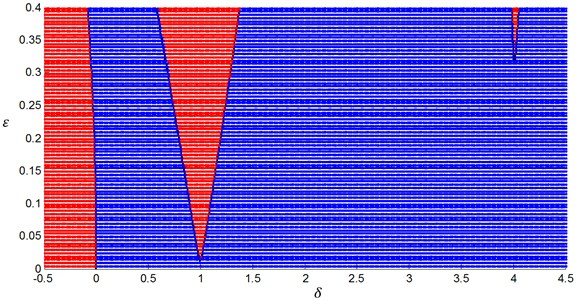### 4.2. Effects of fractional-order parameters on the stability boundaries

In order to illustrate the effects of fractional-order parameters on the stability boundaries, the fractional order $p$ are selected a set of values as 0, 0.2, 0.5, 0.8 and 1 respectively. The transition curves near ${\delta }_{0}=\text{1}$, ${\delta }_{0}=\text{4}$ and ${\delta }_{0}=\text{0}$ are shown in Fig. 2, Fig. 3 and Fig. 4 respectively. From the observation of the changes about the curves in Fig. 2 to Fig. 4, it is found that the increase of the fractional order $p$ could make the ELDC larger, which would result into the upwards moving of the stability boundaries. That is to say, the unstable region becomes smaller and the stable region becomes larger. Simultaneously, it could also be found that the increase of the fractional order $p$ would make the ELSC smaller, which could result into the rightwards moving of the stability boundaries. Therefore, it could be concluded that fractional order $p$ has important influence on the stability boundaries.

Fig. 2The effects of the fractional order p on the stability boundaries for δ0= 1 where ζ= 0.05 and K1= 0.05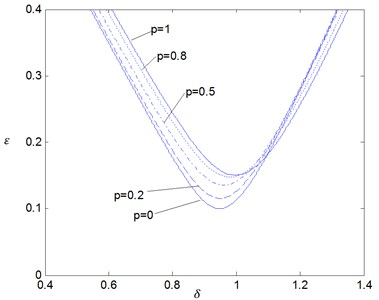Fig. 3The effects of the fractional order p on the stability boundaries for δ0= 4 where ζ= 0.005 and K1= 0.005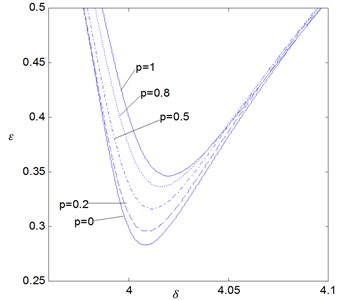Fig. 4The effects of the fractional order p on the stability boundaries for δ0=0 where ζ= 0.5 and K1= 0.5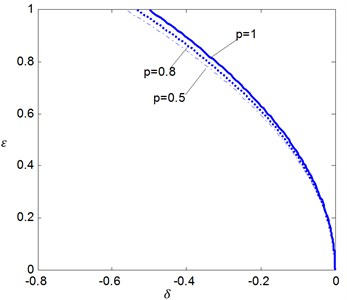Fig. 5The effects of the fractional coefficient K1 on the stability boundaries for δ0= 1 where ζ= 0.05 and p= 0.5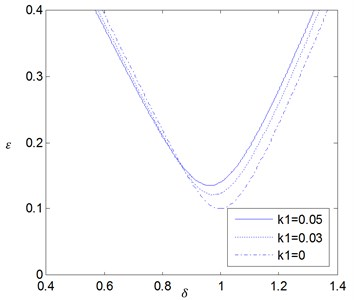Fig. 6The effects of the fractional coefficient K1 on the stability boundaries for δ0= 4 where ζ= 0.005 and p= 0.5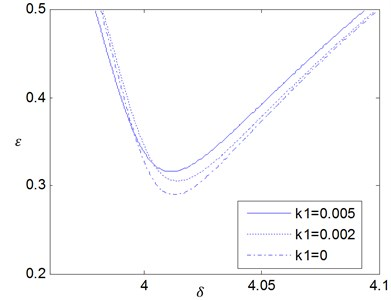Fig. 7The effects of the fractional coefficient K1 on the stability boundaries for δ0=0 where p= 0.5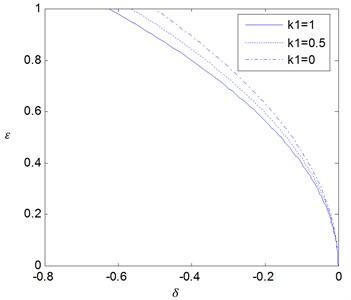The fractional coefficient ${K}_{1}$ are selected some other different values, and the results are shown in Fig. 5 to Fig. 7. From the observation of those curves in Fig. 5 to Fig. 7, it is found that the increase of the fractional coefficient ${K}_{1}$ could make the ELSC larger, which would move the transition curves to the left. Simultaneously, it could be also found that the increase of the fractional coefficient ${K}_{1}$ will make the ELDC lager, which could move the transition curves to the upwards and make the unstable region smaller. Therefore, it could be concluded that the fractional coefficient ${K}_{1}$ has also important influence on the stability boundaries.

## 5. Conclusions

The dynamical characteristics of Mathieu equation with fractional-order derivative is studied by the Lindstedt-Poincare method and the multiple-scale method. The stability boundaries and the corresponding periodic solutions on these boundaries for the constant stiffness ${\delta }_{0}={n}^{2}$ ($n=$ 0, 1, 2, …), are analytically obtained. The effects of the fractional-order parameters on the stability boundaries and the corresponding periodic solutions, including the fractional coefficient and the fractional order, are characterized by the equivalent linear damping coefficient (ELDC) and the equivalent linear stiffness coefficient (ELSC). The comparisons between the transition curves on the boundaries obtained by the approximate analytical solution and the numerical method verify the correctness and satisfactory precision of the analytical solution. It has been illustrated that the different fractional-order parameters have important effects on the stability boundaries. These results are very helpful to design, analyze or control this kind of system, and could present beneficial reference to the similar fractional-order system.

01 February 2015
Accepted
11 April 2015
Published
15 August 2015
SUBJECTS
Chaos, nonlinear dynamics and applications
Keywords
fractional-order derivative
Mathieu equation
Lindstedt-Poincare method
multiple-scale method
stability boundaries
Acknowledgements

The authors are grateful to the support by National Natural Science Foundation of China (No. 11372198), the Program for New Century Excellent Talents in University (NCET-11-0936), the Cultivation Plan for Innovation Team and Leading Talent in Colleges and Universities of Hebei Province (LJRC018), the Program for Advanced Talent in the Universities of Hebei Province (GCC2014053), and the Program for Advanced Talent in Hebei (A201401001).